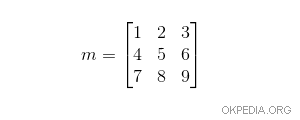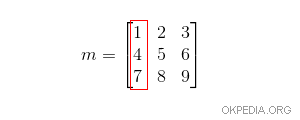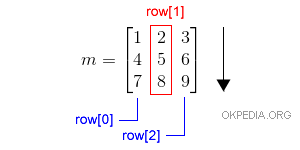# How to extract a column from a matrix in Python

To read a column of a matrix object, previously created with the instruction array() of numpy, you can scroll the elements in the rows of the matrix or transpose the matrix.

Example

m=array([[1,2,3],[4,5,6],[7,8,9]])

This object is equivalent to a 3x3 matrix (3 rows x 3 columns)Let's try to extract the first column with Python.

This problem has the following solutions:

## Solution 1

The matrix can be transposed

m.T

The previous instruction creates an object with columns organized in lists

It is the transposed matrix of m with lines instead of columns.

array([[1, 4, 7],
[2, 5, 8],
[3, 6, 9]])

Now, to read the first column, you can simply extract the first row of the transposed matrix.

The first column is the number 0.

m.T

The command returns the elements of the first column of the matrix.

array([1, 4, 7])

It is the first column of the matrix m.## Solution 2

To select a column of the matrix you can select the elements of the i-th column by scrolling the rows.

This instruction scrolls through all the rows of the matrix m and reads the second element of each using the row function.

[row for row in m]

The output result is as follows:

[2, 5, 8]

It is the second column of the initial matrix.Similarly, the third column can be read by row .

The firest column can be read by row.

Note. The program must read all the rows of the matrix to extract a single column.

https://how.okpedia.org/en/python/how-to-extract-matrix-column-in-pythonReport us an error or send a suggestion to improve this page

Matrix in Python